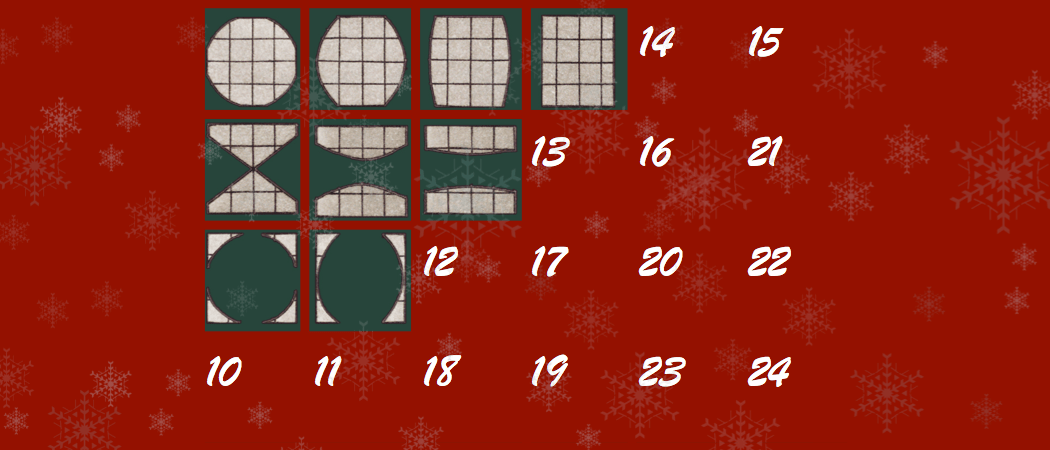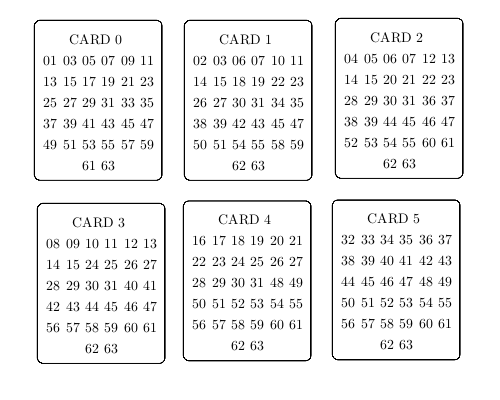# Binary magic card trick

Behind today’s door… A binary magic card trick!This post was part of the Chalkdust 2016 Advent Calendar.

Ah, the Christmas holidays. A time to be spent hiding from the cold, wearing pyjamas, eating too much food and solving integro-differential equations. (At least that’s how I think everyone spends Christmas, right?) Come Christmas Day, after you’ve finally cracked Chalkdust Issue 4’s Crossnumber and stuffed your face with turkey, why don’t you stun your (slightly drunk) family and friends with a very simple math-based magic trick?

### The Trick

This trick follows a fairly standard magic setup: you are going to successfully guess (telepathically, of course) a number decided in secret by the unsuspecting watcher. Hand your target a set of cards with numbers on (an example set of cards can be seen below):Tell them to think of a number in between the smallest and largest number present on the cards (in our case, between 1 and 63). Then ask they hand you every single card with that number on it. Add up the first number on each of the cards given to you, et voilà, you’ve ‘magically’ obtained their number.

### Why does it work?

Afterwards, you’ll be asked how it works. Spend a good bit of time insisting you’re in touch with the Other Side, were recently struck by lightning and can now hear other peoples’ thoughts, or are simply VERY good at guessing. But since, as mathematicians, we need to try and convince people maths is interesting and, more importantly, not a form of witchcraft, consider explaining to them how it works (I’ve found talking over the television during the Christmas edition of Eastenders particularly effective).

Those of you with a keen eye will have clocked it already. Certainly, a big hint is that the first number on each card is a power of 2. The cards have been designed such that each combination of cards uniquely represents a number in binary.

### Binary

For those of you who are not sure what binary is, it is the base two numeral system. You will certainly be familiar with the base ten numeral system since that’s how we normally represent numbers. A good explanation on base systems can be found here.

As an example, we can represent forty-seven in binary and base-ten as

\begin{eqnarray}
\text{forty seven} &=& 4 \times 10^1 + 7 \times 10^0 \\
&=& 47 \,\,\,\, (\text{base ten}) \\
&=& 1 \times 2^5 + 0 \times 2^4 + 1 \times 2^3 + 1 \times 2^2 + 1 \times 2^1 + 1 \times 2^0 \\
&=& 101111 \,\,\,\, (\text{base two})
\end{eqnarray}

So, let us re-order and label the cards as followsCard $N$ is characterised as containing all the numbers which contain a “$1 \times$” in front of the term $2^N$. Taking our example 47 (base ten), we see that 47 appears on cards [0,1,2,3,5]. That is the say

\begin{eqnarray}
47 \,\,\, \text{(base 10)} &=& 1 \times 2^5 + 0 \times 2^4 + 1 \times 2^3 + 1 \times 2^2 + 1 \times 2^1 + 1 \times 2^0 \\
&=& 101111 (\text{base two})
\end{eqnarray}

Ensuring that card $N$ has $2^N$ as the first number on the card (for your ease of reference), simply adding all the first digits on the cards handed back to you will give the correct answer.

We chose 63 as it is of the form $2^6 – 1$. It is best advised to choose a number of the form $2^M -1$ as your maximum number as it ensures each card has $M/2$ digits on it, raising the least suspicion.Alex Doak is a PhD student at UCL researching fluid mechanics.
alexander.doak.11@ucl.ac.uk